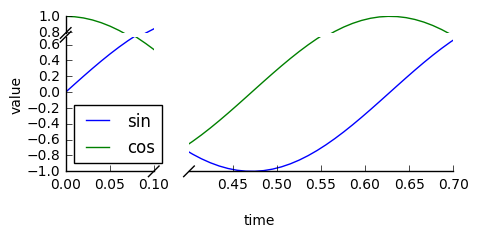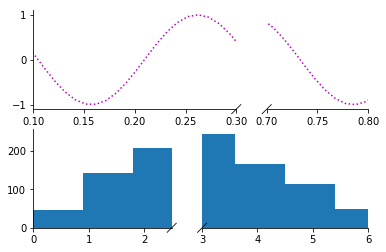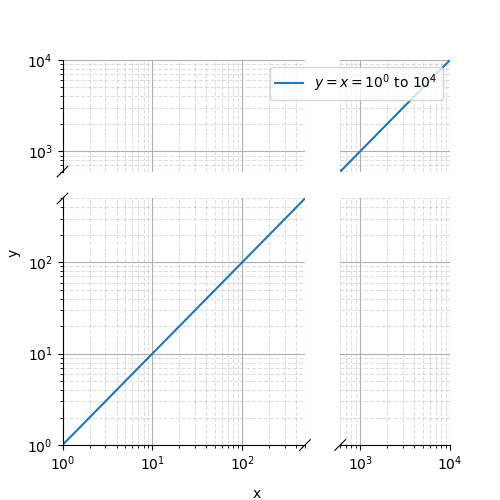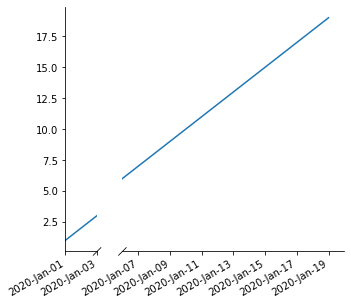Showing:

0

322

1mo ago

8

2

# brokenaxesbrokenaxes makes matplotlib plots with breaks in the axes for showing data across a discontinuous range.

### Features

• Break x and y axes.
• Supports multiple breaks on a single axis.
• Automatically scales axes according to relative ranges.
• Plot multiple lines.
• Legend with positioning relative to entire broken axes object
• x and y label centered to entire plot
• Make brokenaxes object a subplot itself with `matplotlib.GridSpec.subplot_spec`.
• xlims and ylims may be `datetime.datetime` objects
• Supports log scales.

## Installation

I recommend the Anaconda python distribution and this package is available via pypi:

``````pip install brokenaxes
``````

## Usage

``````import matplotlib.pyplot as plt
from brokenaxes import brokenaxes
import numpy as np

fig = plt.figure(figsize=(5, 2))
bax = brokenaxes(xlims=((0, .1), (.4, .7)), ylims=((-1, .7), (.79, 1)), hspace=.05)
x = np.linspace(0, 1, 100)
bax.plot(x, np.sin(10 * x), label='sin')
bax.plot(x, np.cos(10 * x), label='cos')
bax.legend(loc=3)
bax.set_xlabel('time')
bax.set_ylabel('value')
``````### Create subplots

``````from brokenaxes import brokenaxes
from matplotlib.gridspec import GridSpec
import numpy as np

sps1, sps2 = GridSpec(2,1)

bax = brokenaxes(xlims=((.1, .3), (.7, .8)), subplot_spec=sps1)
x = np.linspace(0, 1, 100)
bax.plot(x, np.sin(x*30), ls=':', color='m')

x = np.random.poisson(3, 1000)
bax = brokenaxes(xlims=((0, 2.5), (3, 6)), subplot_spec=sps2)
bax.hist(x, histtype='bar')
``````### Log scales

``````import matplotlib.pyplot as plt
from brokenaxes import brokenaxes
import numpy as np

fig = plt.figure(figsize=(5, 5))
bax = brokenaxes(xlims=((1, 500), (600, 10000)),
ylims=((1, 500), (600, 10000)),
hspace=.15, xscale='log', yscale='log')

x = np.logspace(0.0, 4, 100)
bax.loglog(x, x, label='\$y=x=10^{0}\$ to \$10^{4}\$')

bax.legend(loc='best')
bax.grid(axis='both', which='major', ls='-')
bax.grid(axis='both', which='minor', ls='--', alpha=0.4)
bax.set_xlabel('x')
bax.set_ylabel('y')
plt.show()
``````### datetime

``````import matplotlib.pyplot as plt
from brokenaxes import brokenaxes
import numpy as np
import datetime

fig = plt.figure(figsize=(5, 5))
xx = [datetime.datetime(2020, 1, x) for x in range(1, 20)]

yy = np.arange(1, 20)

bax = brokenaxes(
xlims=(
(
datetime.datetime(2020, 1, 1),
datetime.datetime(2020, 1, 3),
),
(
datetime.datetime(2020, 1, 6),
datetime.datetime(2020, 1, 20),
)
)
)

bax.plot(xx, yy)

fig.autofmt_xdate()
[x.remove() for x in bax.diag_handles]
bax.draw_diags()

import matplotlib.dates as mdates
for ax in bax.axs:
ax.xaxis.set_major_formatter(mdates.DateFormatter('%Y-%b-%d'))
``````## How do I do more?

You can customize brokenaxes outside of the supported features listed above. Brokenaxes works by creating a number of smaller axes objects, with the positions and sizes of those axes dictated by the data ranges used in the constructor. Those individual axes are stored as a list in `bax.axs`. Most customizations will require accessing those inner axes objects. (See the last two lines of the datetime example). There is also a larger invisible axes object, `bax.big_ax`, which spans the entire brokenaxes region and is used for things like x and y axis labels which span all of the smaller axes.

If you make a plot with this tool that you are proud of, send me a png and code and I'll add it to the gallery!

Please use this tool wisely. Any data visualization techique can be used to elucidate trends in the data, ben can also be used to manipulate and mislead. The latter is particularly true for broken axes plots, so please try to use them responsibly. Other than that, this software is free to use. See the license file for details.

## Rate & Review

Great Documentation0
Easy to Use0
Performant0
Highly Customizable0
Bleeding Edge0
Responsive Maintainers0
Poor Documentation0
Hard to Use0
Slow0
Buggy0
Abandoned0
Unwelcoming Community0
100